# Class 12 Maths NCERT Solutions for Chapter 13 Probability Exercise 13.1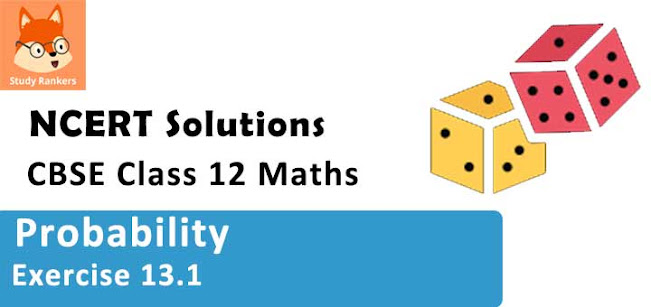### Probability Exercise 13.1 Solutions

1. Given that E and F are events such that P(E) = 0.6, P(F) = 0.3 and P(E∩F) = 0.2, find P (E|F) and P(F|E).

Solution

It is given that P(E) = 0.6, P(F) = 0.3, and P(E⋂F) 0.2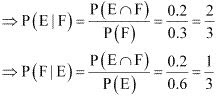2. Compute P(A|B), if P(B) = 0.5 and P (A ∩ B) = 0.32

Solution

It is given that P(B) = 0.5 and P(A ∩ B) = 0.32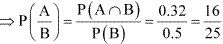3. If P(A) = 0.8, P(B) = 0.5 and P(B|A) = 0.4, find
(i) P(A ∩ B)
(ii) P(A|B)
(iii) P(A ∪ B)

Solution

It is given that P(A) = 0.8, P(B) = 0.5, and P(B|A) = 0.4
(i) P (B|A) = 0.4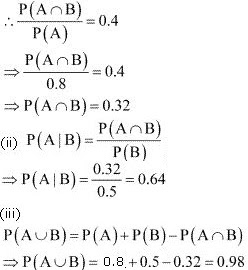4. Evaluate P (A ∪ B), if 2P (A) = P (B) =5/13 and P(A|B) =2/5

Solution

It is given that, 2P(A) = P(B) = 5/13
⇒ P(A) = 5/26 and P(B) = 5/13
P(A/B) = 2/5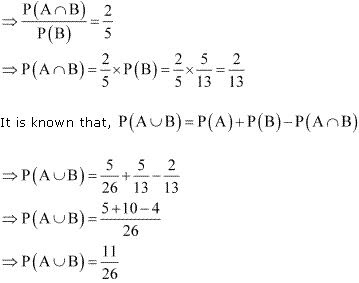5. If P(A) = 6/11, P(B) = 5/11 and and P(A ∪ B) = 7/11 find
(i) P(A ∩ B)
(ii) P(A|B)
(iii) P(B|A)

Solution

It is given that P(A) = 6/11, P(B) = 5/11, and P(A ∪ B) = 7/11
(i) P(A ∪ B) = 7/11
∴ P(A) + P(B) - P(A ∩ B) = 7/11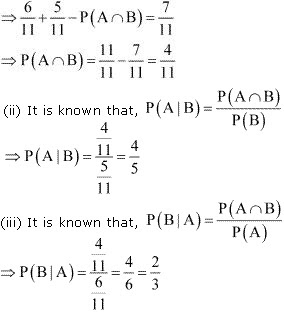6. A coin is tossed three times, where
(i) E: head on third toss, F: heads on first two tosses
(iii) E: at most two tails, F: at least one tail

Solution

If a coin is tossed three times, then the sample space S is
S = {HHH, HHT, HTH, HTT, THH, THT, TTH, TTT}
It can be seen that the sample space has 8 elements.
(i) E = {HHH, HTH, THH, TTH}
F = {HHH, HHT}E ∩ F = {HHH}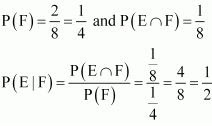(ii) E = {HHH, HHT, HTH, THH}
F = {HHT, HTH, HTT, THH, THT, TTH, TTT}E ∩ F = {HHT, HTH, THH}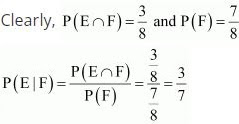(iii) E = {HHH, HHT, HTT, HTH, THH, THT, TTH}
F = {HHT, HTT, HTH, THH, THT, TTH, TTT}
∴ E ∩ F = { HHT, HTT, HTH, THH, THT, TTH}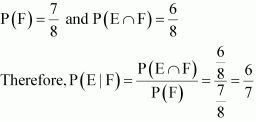7. Two coins are tossed once, where
(i) E: tail appears on one coin, F: one coin shows head
(ii) E: not tail appears, F: no head appears

Solution

If two coins are tossed once, then the sample space S is
S = { HH, HT, TH, TT}
(i) E = { HT, TH}
F = { HT, TH}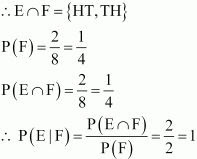(ii) E = {HH}
F = {TT}
∴ E ∩ F = Φ
P (F) = 1 and P (E ∩ F) = 08. A die is thrown three times, E: 4 appears on the third toss, F: 6 and 5 appears respectively on first two tosses .

Solution

If a die is thrown three times, then the number of elements in the sample space will be 6 × 6 × 6 = 216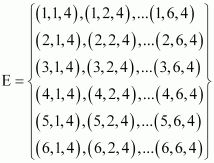F = {(6, 5, 1), (6, 5, 2), (6, 5, 3), (6, 5, 4), (6, 5, 5), (6, 5, 6)}
∴ E ∩ F = {(6, 5, 4)}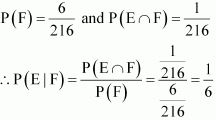9. Mother, father and son line up at random for a family picture
E: son on one end, F: father in middle

Solution

If mother (M), father (F), and son (S) line up for the family picture, then the sample space will be
S = {MFS, MSF, FMS, FSM, SMF, SFM}
⇒ E = {MFS, FMS, SMF, SFM}
F = {MFS, SFM}
∴ E ∩ F = {MFS, SFM}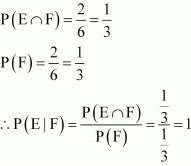10. A black and a red dice are rolled.
(a) Find the conditional probability of obtaining a sum greater than 9, given that the black die resulted in a 5.
(b) Find the conditional probability of obtaining the sum 8, given that the red die resulted in a number less than 4.

Solution

Let the first observation be from the black die and second from the red die.
When two dice (one black and another red) are rolled, the sample space S has 6 × 6 = 36 number of elements.
Let
A: Obtaining a sum greater than 9
= {(4, 6), (5, 5), (5, 6), (6, 4), (6, 5), (6, 6)}
B: Black die results in a 5.
= {(5, 1), (5, 2), (5, 3), (5, 4), (5, 5), (5, 6)}
∴ A ∩ B = {(5, 5), (5, 6)}
The conditional probability of obtaining a sum greater than 9, given that the black die resulted in a 5, is given by P (A|B).
PA|B = PA∩BPB = 236636 = 26 = 13
(b) E: Sum of the observations is 8.
= {(2, 6), (3, 5), (4, 4), (5, 3), (6, 2)}
F: Red die resulted in a number less than 4.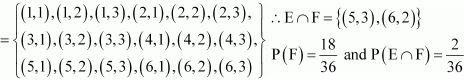The conditional probability of obtaining the sum equal to 8, given that the red die resulted in a number less than 4, is given by P (E/F).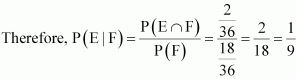11. A fair die is rolled. Consider events E = {1, 3, 5}, F = {2, 3} and G = {2, 3, 4, 5}
Find
(i) P (E|F) and P (F|E)
(ii) P (E|G) and P (G|E)
(ii) P ((E ∪ F)|G) and P ((E ∩ G)|G)

Solution

When a fair die is rolled, the sample space S will be
S = {1, 2, 3, 4, 5, 6}
It is given that E = {1, 3, 5}, F = {2, 3}, and G = {2, 3, 4, 5}
∴ P(E) = 3/6 = 1/2
P(F) = 2/6 = 1/3
P(G) = 4/6 = 2/3
(i) E ∩ F = {3}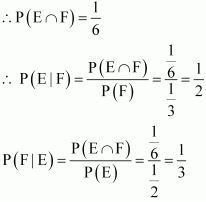(ii) E ∩ G = {3, 5}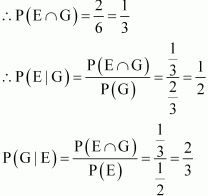(iii) E ∪ F = {1, 2, 3, 5}
(E ∪ F) ∩ G = {1, 2, 3, 5} ∩{2, 3, 4, 5} = {2, 3, 5}
E ∩ F = {3}
(E ∩ F) ∩ G = {3}∩{2, 3, 4, 5} = {3}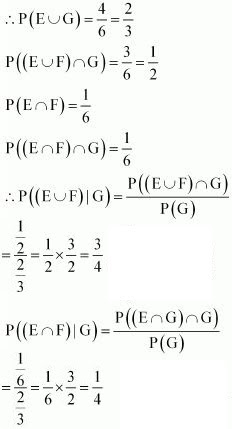12. Assume that each born child is equally likely to be a boy or a girl. If a family has two children, what is the conditional probability that both are girls given that
(i) the youngest is a girl,
(ii) at least one is a girl?

Solution

Let b and g represent the boy and the girl child respectively. If a family has two children, the sample space will be
S = {(bb), (bg), (gb), (g, g)}
Let A be the event that both children are girls.
∴ A = {(g, g)}
(i) Let B be the event that the youngest child is a girl.
∴ B = [(b, g), (g, g)]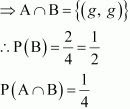The conditional probability that both are girls, given that the youngest child is a girl, is given by P (A|B).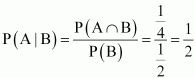Therefore, the required probability is 1/2.
(ii) Let C be the event that at least one child is a girl.
∴ C = {(b, g), (g, b), (g, g)}
⇒ A ∩ C = { g, g}
⇒ P(C) = 3/4
P(A∩C) = 1/4
The conditional probability that both are girls, given that at least one child is a girl, is given by P(A/C).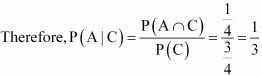13. An instructor has a question bank consisting of 300 easy True/False questions, 200 difficult True/False questions, 500 easy multiple choice questions and 400 difficult multiple choice questions. If a question is selected at random from the question bank, what is the probability that it will be an easy question given that it is a multiple choice question?

Solution

The given data can be tabulated as

 True/False Multiple choice Total Easy 300 500 800 Difficult 200 400 600 Total 500 900 1400

Let us denote E = easy questions, M = multiple choice questions, D = difficult questions, and T = True/False questions
Total number of questions = 1400
Total number of multiple choice questions = 900
Therefore, probability of selecting an easy multiple choice question is
P (E ∩ M) = 500/1400 = 5/14
Probability of selecting a multiple choice question, P (M), is 900/1400 = 9/14
P (E|M) represents the probability that a randomly selected question will be an easy question, given that it is a multiple choice question.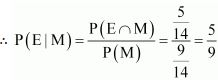Therefore, the required probability is 5/9.

14. Given that the two numbers appearing on throwing the two dice are different. Find the probability of the event ‘the sum of numbers on the dice is 4’.

Solution

When dice is thrown, number of observations in the sample space = 6 × 6 = 36
Let A be the event that the sum of the numbers on the dice is 4 and B be the event that the two numbers appearing on throwing the two dice are different. ∴ A = {(1, 3), (2, 2), (3, 1)}
B=1, 21, 31, 41, 51, 62, 12, 32, 42, 52, 63, 13, 23, 43, 53, 64, 14, 24, 34, 54, 65, 15, 25, 35, 45,66, 16, 26, 36, 46, 5 A∩B = 1, 3, 3, 1
∴ P(B) = 3036 =56 and
PA∩B = 236 = 118Let P (A|B) represent the probability that the sum of the numbers on the dice is 4, given that the two numbers appearing on throwing the two dice are different.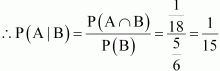Therefore, the required probability is 1/15.

15. Consider the experiment of throwing a die, if a multiple of 3 comes up, throw the die again and if any other number comes, toss a coin. Find the conditional probability of the event ‘the coin shows a tail’, given that ‘at least one die shows a 3’.

Solution

The outcomes of the given experiment can be represented by the following tree diagram.
The sample space of the experiment is,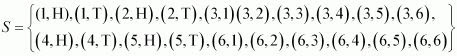Let A be the event that the coin shows a tail and B be the event that at least one die show 3.
∴ A = { (1,T), (2, T), (4, T) , (5, T)}
B = {(3, 1), (3, 2), (3, 3), (3, 4),
⇒ A ∩ B = ∅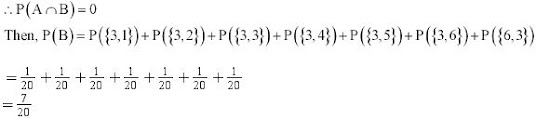Probability of the event that the coin shows a tail, given that at least one die shows 3, is given by P(A|B).
Therefore,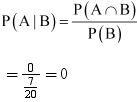16. If P(A) = 1/2, P(B) = 0, then P(A|B) is
(A) 0
(B) 1/2
(C) not defined
(D) 1

Solution

It is given that P(A) = 1/2 and P(B) = 0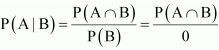Therefore, P(A/B) is not defined.
Thus, the correct answer is C.

17. If P(A) = 1/2, P(B) = 0, then P(A|B) is
(A) 0
(B) 1/2
(C) not defined
(D) 1

Solution

It is given that, P(A|B) = P(B|A)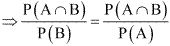P(A) = P(B)
Thus, the correct answer is D.